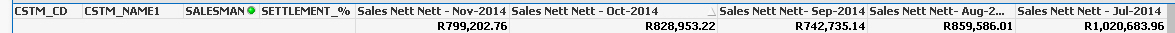# QlikView App Dev

Discussion Board for collaboration related to QlikView App Development.

Announcements
QlikWorld online is next week! REGISTER NOW
cancel
Showing results for
Did you mean:Not applicable

## Expression totals change when selecting different month

Hi,

i Have a report with 3 dimensions and 4 Expressions to calculate the "nett nett" for the last four months.

when i choose a different month the total changes. ie Column 1 was Sep , now column 2 is Sep but total is different

below image is SEP as first month in Column1now i selected oct as month which moves sep to 2nd column total differs.now when i select nov both change oct and sep. as belowcolumn 1 expression

=(sum({<MonthYear = {"\$(=MaxString(MonthYear))"}>} Price_Lens_Total_Net)

+sum({<MonthYear = {"\$(=MaxString(MonthYear))"}>} Price_Semi_Net)

+sum({<MonthYear = {"\$(=MaxString(MonthYear))"}>}Price_Frame_Net))* SETTLEMENT_%

Column2 expression

+sum({<Month =,MonthYear = {"\$(=Date(AddMonths(Max(OrderDate),-1),'MMM-YYYY'))"}>} Price_Frame_Net))* SETTLEMENT_%

Column3 expression

* SETTLEMENT_%

i am multiplying the totals with the settlement discount precentage.

i have the totals mode set on sum of rows on all expressions.

any help in the right direction would be appreciated.

thank you

Kallie

1 Solution

Accepted SolutionsMVP

SETTLEMENT_% is a naked aggregation. Qlikview actually calculates only(SETTLEMENT_%). And that expression does not include the set modifiers you added to the sums. So your selections do influence the value of SETTLEMENT_% that is used in the calculations. Try adding a set modifier to only(SETTLEMENT_%) too.

talk is cheap, supply exceeds demand
2 RepliesMVP

SETTLEMENT_% is a naked aggregation. Qlikview actually calculates only(SETTLEMENT_%). And that expression does not include the set modifiers you added to the sums. So your selections do influence the value of SETTLEMENT_% that is used in the calculations. Try adding a set modifier to only(SETTLEMENT_%) too.

talk is cheap, supply exceeds demandNot applicable
Author

thank you that solves it